Victor Hui

•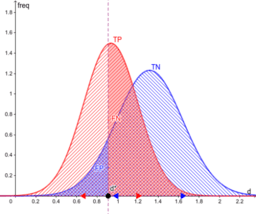ROC Curve

Activity

Victor Hui

•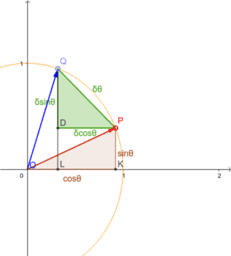Derivatives of sine and cosine

Activity

Victor Hui

•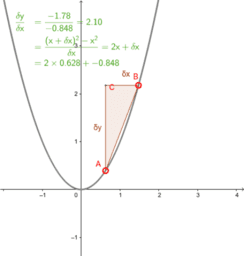The idea of limit in differential calculus

Activity

Victor Hui

•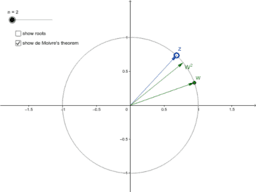Roots of a complex number

Activity

Victor Hui

•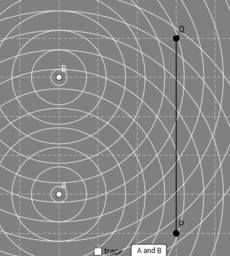Young's double slit experiment

Activity

Victor Hui

•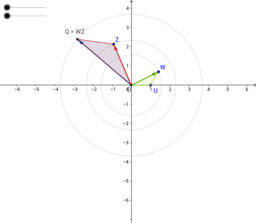Multiplication of two complex numbers

Activity

Victor Hui

•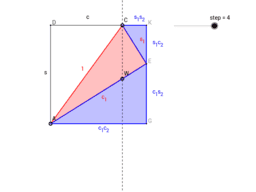Sine and Cosine of Two Angles

Activity

Victor Hui

•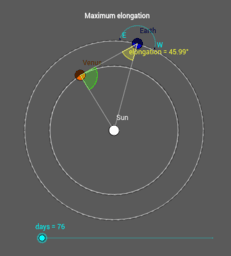Observing Venus

Activity

Victor Hui

•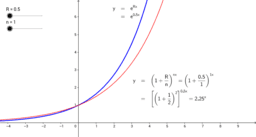"e" as the limit of a binomial

Activity

Victor Hui

•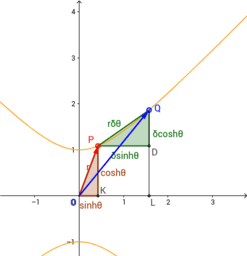Derivatives of hyperbolic sine and cosine

Activity

Victor Hui

•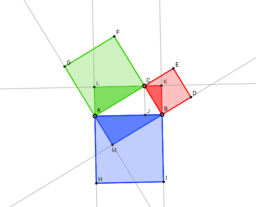The Pythagorean theorem and the Euclidean plane (2)

Activity

Victor Hui

•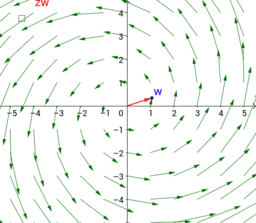Transformation of complex numbers

Activity

Victor Hui

•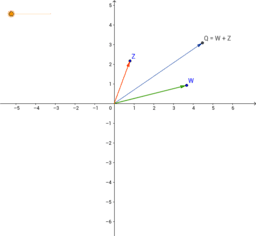Activity

Victor Hui

•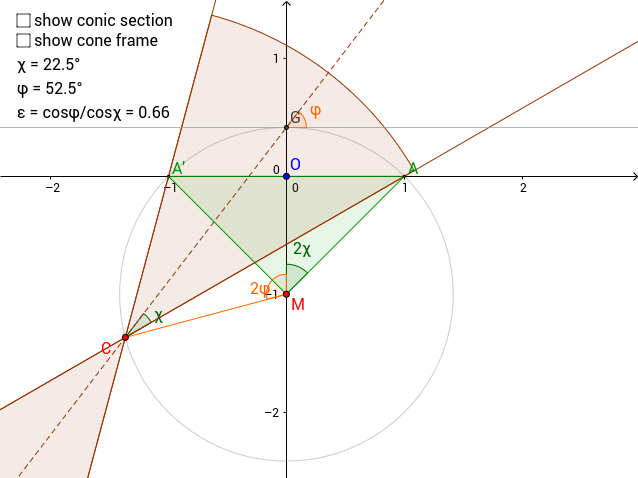Planar cross-sections of a cone

Activity

Victor Hui

•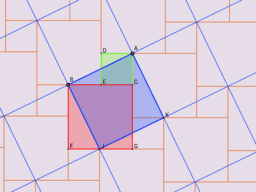The Pythagorean theorem and the Euclidean plane

Activity

Victor Hui

•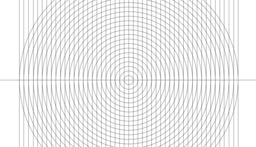Conic sections

Book

Victor Hui

•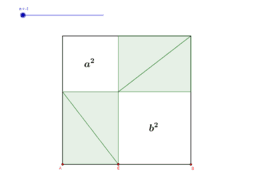Pythagoras

Book

Victor Hui

•Calculus

Book

Victor Hui

•Complex numbers

Book

Victor Hui

•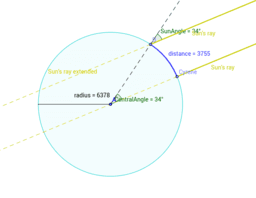Astronomy

Book

Victor Hui

•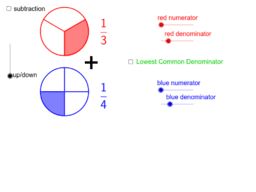Book

Victor Hui